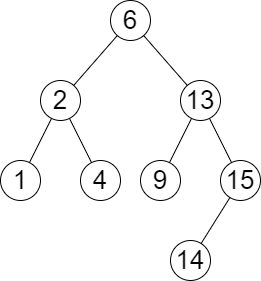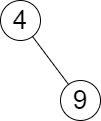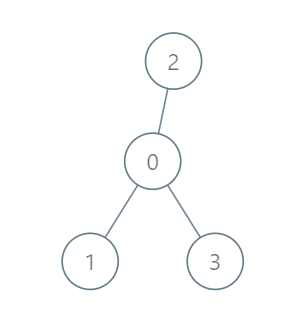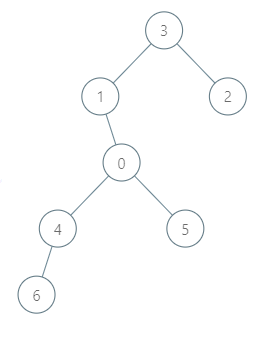# weekly-contest-320

## A

### Statement

• `0 <= i < j < k < nums.length`
• `nums[i]``nums[j]``nums[k]` 两两不同
• 换句话说：`nums[i] != nums[j]``nums[i] != nums[k]``nums[j] != nums[k]`

``````输入：nums = [4,4,2,4,3]

- (0, 2, 4) 因为 4 != 2 != 3
- (1, 2, 4) 因为 4 != 2 != 3
- (2, 3, 4) 因为 2 != 4 != 3

``````

``````输入：nums = [1,1,1,1,1]

``````

• `3 <= nums.length <= 100`
• `1 <= nums[i] <= 1000`

You are given a 0-indexed array of positive integers `nums`. Find the number of triplets `(i, j, k)` that meet the following conditions:

• `0 <= i < j < k < nums.length`
• `nums[i]`, `nums[j]`, and `nums[k]` are pairwise distinct.
• In other words, `nums[i] != nums[j]`, `nums[i] != nums[k]`, and `nums[j] != nums[k]`.

Return the number of triplets that meet the conditions.

Example 1:

``````Input: nums = [4,4,2,4,3]
Output: 3
Explanation: The following triplets meet the conditions:
- (0, 2, 4) because 4 != 2 != 3
- (1, 2, 4) because 4 != 2 != 3
- (2, 3, 4) because 2 != 4 != 3
Since there are 3 triplets, we return 3.
Note that (2, 0, 4) is not a valid triplet because 2 > 0.
``````

Example 2:

``````Input: nums = [1,1,1,1,1]
Output: 0
Explanation: No triplets meet the conditions so we return 0.
``````

Constraints:

• `3 <= nums.length <= 100`
• `1 <= nums[i] <= 1000`

### Solution

``````#include <bits/stdc++.h>
#include <ext/pb_ds/assoc_container.hpp>
#include <ext/pb_ds/tree_policy.hpp>

#define endl "\n"
#define fi first
#define se second
#define all(x) begin(x), end(x)
#define rall rbegin(a), rend(a)
#define bitcnt(x) (__builtin_popcountll(x))
#define complete_unique(a) a.erase(unique(begin(a), end(a)), end(a))
#define mst(x, a) memset(x, a, sizeof(x))
#define MP make_pair

using ll = long long;
using ull = unsigned long long;
using db = double;
using ld = long double;
using VLL = std::vector<ll>;
using VI = std::vector<int>;
using PII = std::pair<int, int>;
using PLL = std::pair<ll, ll>;

using namespace __gnu_pbds;
using namespace std;
template <typename T>
using ordered_set = tree<T, null_type, less<T>, rb_tree_tag, tree_order_statistics_node_update>;

template <typename T, typename S>
inline bool chmax(T &a, const S &b) {
return a < b ? a = b, 1 : 0;
}

template <typename T, typename S>
inline bool chmin(T &a, const S &b) {
return a > b ? a = b, 1 : 0;
}

#ifdef LOCAL
#include <debug.hpp>
#else
#define dbg(...)
#endif

class Solution {
public:
int unequalTriplets(vector<int> &nums) {
int n = int(nums.size());

int res = 0;

for (int i = 0; i < n; i++) {
for (int j = i + 1; j < n; j++) {
for (int k = j + 1; k < n; k++) {
int a = nums[i];
int b = nums[j];
int c = nums[k];

if (a != b && b != c && a != c) {
++res;
}
}
}
}

return res;
}
};

#ifdef LOCAL

int main() {
return 0;
}

#endif
``````

## B

### Statement

• `mini` 是树中小于等于 `queries[i]`最大值 。如果不存在这样的值，则使用 `-1` 代替。
• `maxi` 是树中大于等于 `queries[i]`最小值 。如果不存在这样的值，则使用 `-1` 代替。``````输入：root = [6,2,13,1,4,9,15,null,null,null,null,null,null,14], queries = [2,5,16]

- 树中小于等于 2 的最大值是 2 ，且大于等于 2 的最小值也是 2 。所以第一个查询的答案是 [2,2] 。
- 树中小于等于 5 的最大值是 4 ，且大于等于 5 的最小值是 6 。所以第二个查询的答案是 [4,6] 。
- 树中小于等于 16 的最大值是 15 ，且大于等于 16 的最小值不存在。所以第三个查询的答案是 [15,-1] 。
````````````输入：root = [4,null,9], queries = 

``````

• 树中节点的数目在范围 `[2, 105]`
• `1 <= Node.val <= 106`
• `n == queries.length`
• `1 <= n <= 105`
• `1 <= queries[i] <= 106`

You are given the `root` of a binary search tree and an array `queries` of size `n` consisting of positive integers.

Find a 2D array `answer` of size `n` where `answer[i] = [mini, maxi]`:

• `mini` is the largest value in the tree that is smaller than or equal to `queries[i]`. If a such value does not exist, add `-1` instead.
• `maxi` is the smallest value in the tree that is greater than or equal to `queries[i]`. If a such value does not exist, add `-1` instead.

Return the array `answer`.

Example 1:``````Input: root = [6,2,13,1,4,9,15,null,null,null,null,null,null,14], queries = [2,5,16]
Output: [[2,2],[4,6],[15,-1]]
Explanation: We answer the queries in the following way:
- The largest number that is smaller or equal than 2 in the tree is 2, and the smallest number that is greater or equal than 2 is still 2. So the answer for the first query is [2,2].
- The largest number that is smaller or equal than 5 in the tree is 4, and the smallest number that is greater or equal than 5 is 6. So the answer for the second query is [4,6].
- The largest number that is smaller or equal than 16 in the tree is 15, and the smallest number that is greater or equal than 16 does not exist. So the answer for the third query is [15,-1].
``````

Example 2:``````Input: root = [4,null,9], queries = 
Output: [[-1,4]]
Explanation: The largest number that is smaller or equal to 3 in the tree does not exist, and the smallest number that is greater or equal to 3 is 4. So the answer for the query is [-1,4].
``````

Constraints:

• The number of nodes in the tree is in the range `[2, 105]`.
• `1 <= Node.val <= 106`
• `n == queries.length`
• `1 <= n <= 105`
• `1 <= queries[i] <= 106`

### Solution

``````#include <bits/stdc++.h>
#include <ext/pb_ds/assoc_container.hpp>
#include <ext/pb_ds/tree_policy.hpp>

#define endl "\n"
#define fi first
#define se second
#define all(x) begin(x), end(x)
#define rall rbegin(a), rend(a)
#define bitcnt(x) (__builtin_popcountll(x))
#define complete_unique(a) a.erase(unique(begin(a), end(a)), end(a))
#define mst(x, a) memset(x, a, sizeof(x))
#define MP make_pair

using ll = long long;
using ull = unsigned long long;
using db = double;
using ld = long double;
using VLL = std::vector<ll>;
using VI = std::vector<int>;
using PII = std::pair<int, int>;
using PLL = std::pair<ll, ll>;

using namespace __gnu_pbds;
using namespace std;
template <typename T>
using ordered_set = tree<T, null_type, less<T>, rb_tree_tag, tree_order_statistics_node_update>;

template <typename T, typename S>
inline bool chmax(T &a, const S &b) {
return a < b ? a = b, 1 : 0;
}

template <typename T, typename S>
inline bool chmin(T &a, const S &b) {
return a > b ? a = b, 1 : 0;
}

#ifdef LOCAL
#include <debug.hpp>
#else
#define dbg(...)
#endif

/**
* Definition for a binary tree node.
* struct TreeNode {
*     int val;
*     TreeNode *left;
*     TreeNode *right;
*     TreeNode() : val(0), left(nullptr), right(nullptr) {}
*     TreeNode(int x) : val(x), left(nullptr), right(nullptr) {}
*     TreeNode(int x, TreeNode *left, TreeNode *right) : val(x), left(left), right(right) {}
* };
*/

#ifdef LOCAL

struct TreeNode {
int val;
TreeNode *left;
TreeNode *right;
TreeNode() : val(0), left(nullptr), right(nullptr) {}
TreeNode(int x) : val(x), left(nullptr), right(nullptr) {}
TreeNode(int x, TreeNode *left, TreeNode *right) : val(x), left(left), right(right) {}
};

#endif

class Solution {
public:
vector<int> a;
void dfs(TreeNode *rt) {
if (!rt) {
return;
}

a.push_back(rt->val);
dfs(rt->left);
dfs(rt->right);
}

vector<vector<int>> closestNodes(TreeNode *root, vector<int> &queries) {
a.clear();
dfs(root);

sort(all(a));

auto res = vector<vector<int>>();

for (const auto &q : queries) {
auto pos = lower_bound(all(a), q);
int mi = -1;
int mx = -1;

if (pos == a.end()) {
--pos;
mi = *pos;
} else {
mx = *pos;
if (mx == q) {
mi = mx;
} else if (pos != a.begin()) {
--pos;
mi = *pos;
}
}

res.push_back({mi, mx});
}

return res;
}
};

#ifdef LOCAL

int main() {
return 0;
}

#endif
``````

## C

### Statement``````输入：roads = [[0,1],[0,2],[0,3]], seats = 5

- 代表 1 直接到达首都，消耗 1 升汽油。
- 代表 2 直接到达首都，消耗 1 升汽油。
- 代表 3 直接到达首都，消耗 1 升汽油。

````````````输入：roads = [[3,1],[3,2],[1,0],[0,4],[0,5],[4,6]], seats = 2

- 代表 2 到达城市 3 ，消耗 1 升汽油。
- 代表 2 和代表 3 一起到达城市 1 ，消耗 1 升汽油。
- 代表 2 和代表 3 一起到达首都，消耗 1 升汽油。
- 代表 1 直接到达首都，消耗 1 升汽油。
- 代表 5 直接到达首都，消耗 1 升汽油。
- 代表 6 到达城市 4 ，消耗 1 升汽油。
- 代表 4 和代表 6 一起到达首都，消耗 1 升汽油。

````````````输入：roads = [], seats = 1

``````

• `1 <= n <= 105`
• `roads.length == n - 1`
• `roads[i].length == 2`
• `0 <= ai, bi < n`
• `ai != bi`
• `roads` 表示一棵合法的树。
• `1 <= seats <= 105`

There is a tree (i.e., a connected, undirected graph with no cycles) structure country network consisting of `n` cities numbered from `0` to `n - 1` and exactly `n - 1` roads. The capital city is city `0`. You are given a 2D integer array `roads` where `roads[i] = [ai, bi]` denotes that there exists a bidirectional road connecting cities `ai` and `bi`.

There is a meeting for the representatives of each city. The meeting is in the capital city.

There is a car in each city. You are given an integer `seats` that indicates the number of seats in each car.

A representative can use the car in their city to travel or change the car and ride with another representative. The cost of traveling between two cities is one liter of fuel.

Return the minimum number of liters of fuel to reach the capital city.

Example 1:``````Input: roads = [[0,1],[0,2],[0,3]], seats = 5
Output: 3
Explanation:
- Representative1 goes directly to the capital with 1 liter of fuel.
- Representative2 goes directly to the capital with 1 liter of fuel.
- Representative3 goes directly to the capital with 1 liter of fuel.
It costs 3 liters of fuel at minimum.
It can be proven that 3 is the minimum number of liters of fuel needed.
``````

Example 2:``````Input: roads = [[3,1],[3,2],[1,0],[0,4],[0,5],[4,6]], seats = 2
Output: 7
Explanation:
- Representative2 goes directly to city 3 with 1 liter of fuel.
- Representative2 and representative3 go together to city 1 with 1 liter of fuel.
- Representative2 and representative3 go together to the capital with 1 liter of fuel.
- Representative1 goes directly to the capital with 1 liter of fuel.
- Representative5 goes directly to the capital with 1 liter of fuel.
- Representative6 goes directly to city 4 with 1 liter of fuel.
- Representative4 and representative6 go together to the capital with 1 liter of fuel.
It costs 7 liters of fuel at minimum.
It can be proven that 7 is the minimum number of liters of fuel needed.
``````

Example 3:``````Input: roads = [], seats = 1
Output: 0
Explanation: No representatives need to travel to the capital city.
``````

Constraints:

• `1 <= n <= 105`
• `roads.length == n - 1`
• `roads[i].length == 2`
• `0 <= ai, bi < n`
• `ai != bi`
• `roads` represents a valid tree.
• `1 <= seats <= 105`

### Solution

``````#include <bits/stdc++.h>
#include <ext/pb_ds/assoc_container.hpp>
#include <ext/pb_ds/tree_policy.hpp>

#define endl "\n"
#define fi first
#define se second
#define all(x) begin(x), end(x)
#define rall rbegin(a), rend(a)
#define bitcnt(x) (__builtin_popcountll(x))
#define complete_unique(a) a.erase(unique(begin(a), end(a)), end(a))
#define mst(x, a) memset(x, a, sizeof(x))
#define MP make_pair

using ll = long long;
using ull = unsigned long long;
using db = double;
using ld = long double;
using VLL = std::vector<ll>;
using VI = std::vector<int>;
using PII = std::pair<int, int>;
using PLL = std::pair<ll, ll>;

using namespace __gnu_pbds;
using namespace std;
template <typename T>
using ordered_set = tree<T, null_type, less<T>, rb_tree_tag, tree_order_statistics_node_update>;

template <typename T, typename S>
inline bool chmax(T &a, const S &b) {
return a < b ? a = b, 1 : 0;
}

template <typename T, typename S>
inline bool chmin(T &a, const S &b) {
return a > b ? a = b, 1 : 0;
}

#ifdef LOCAL
#include <debug.hpp>
#else
#define dbg(...)
#endif

class Solution {
public:
vector<vector<int>> G;
int seats;
pair<ll, int> dfs(int u, int fa) {
ll cost = 0;
int cnt = 1;

for (const auto &v : G[u]) {
if (v == fa) {
continue;
}

auto p = dfs(v, u);
cost += p.first;
cost += (p.second + seats - 1) / seats;
cnt += p.second;
}

return make_pair(cost, cnt);
}

long long minimumFuelCost(vector<vector<int>> &roads, int seats) {
int n = int(roads.size()) + 1;
G = vector<vector<int>>(n + 1, vector<int>());
this->seats = seats;

for (const auto &r : roads) {
int u = r;
int v = r;
G[u].push_back(v);
G[v].push_back(u);
}

return dfs(0, -1).first;
}
};

#ifdef LOCAL

int main() {
return 0;
}

#endif
``````

## D

### Statement

• `s` 被分成 `k` 段互不相交的子字符串。
• 每个子字符串长度都 至少 为 `minLength` 。
• 每个子字符串的第一个字符都是一个 质数 数字，最后一个字符都是一个 非质数 数字。质数数字为 `'2'` ，`'3'` ，`'5'` 和 `'7'` ，剩下的都是非质数数字。

``````输入：s = "23542185131", k = 3, minLength = 2

"2354 | 218 | 5131"
"2354 | 21851 | 31"
"2354218 | 51 | 31"
``````

``````输入：s = "23542185131", k = 3, minLength = 3

``````

``````输入：s = "3312958", k = 3, minLength = 1

``````

• `1 <= k, minLength <= s.length <= 1000`
• `s` 每个字符都为数字 `'1'` 到 `'9'` 之一。

You are given a string `s` that consists of the digits `'1'` to `'9'` and two integers `k` and `minLength`.

A partition of `s` is called beautiful if:

• `s` is partitioned into `k` non-intersecting substrings.
• Each substring has a length of at least `minLength`.
• Each substring starts with a prime digit and ends with a non-prime digit. Prime digits are `'2'`, `'3'`, `'5'`, and `'7'`, and the rest of the digits are non-prime.

Return the number of beautiful partitions of `s`. Since the answer may be very large, return it modulo `109 + 7`.

A substring is a contiguous sequence of characters within a string.

Example 1:

``````Input: s = "23542185131", k = 3, minLength = 2
Output: 3
Explanation: There exists three ways to create a beautiful partition:
"2354 | 218 | 5131"
"2354 | 21851 | 31"
"2354218 | 51 | 31"
``````

Example 2:

``````Input: s = "23542185131", k = 3, minLength = 3
Output: 1
Explanation: There exists one way to create a beautiful partition: "2354 | 218 | 5131".
``````

Example 3:

``````Input: s = "3312958", k = 3, minLength = 1
Output: 1
Explanation: There exists one way to create a beautiful partition: "331 | 29 | 58".
``````

Constraints:

• `1 <= k, minLength <= s.length <= 1000`
• `s` consists of the digits `'1'` to `'9'`.

### Solution

``````#include <bits/stdc++.h>
#include <ext/pb_ds/assoc_container.hpp>
#include <ext/pb_ds/tree_policy.hpp>

#define endl "\n"
#define fi first
#define se second
#define all(x) begin(x), end(x)
#define rall rbegin(a), rend(a)
#define bitcnt(x) (__builtin_popcountll(x))
#define complete_unique(a) a.erase(unique(begin(a), end(a)), end(a))
#define mst(x, a) memset(x, a, sizeof(x))
#define MP make_pair

using ll = long long;
using ull = unsigned long long;
using db = double;
using ld = long double;
using VLL = std::vector<ll>;
using VI = std::vector<int>;
using PII = std::pair<int, int>;
using PLL = std::pair<ll, ll>;

using namespace __gnu_pbds;
using namespace std;
template <typename T>
using ordered_set = tree<T, null_type, less<T>, rb_tree_tag, tree_order_statistics_node_update>;

template <typename T, typename S>
inline bool chmax(T &a, const S &b) {
return a < b ? a = b, 1 : 0;
}

template <typename T, typename S>
inline bool chmin(T &a, const S &b) {
return a > b ? a = b, 1 : 0;
}

#ifdef LOCAL
#include <debug.hpp>
#else
#define dbg(...)
#endif

class Solution {
public:
inline static constexpr int mod = 1e9 + 7;

inline static constexpr bool is_prime[] = {
0,
0,
1,
1,
0,
1,
0,
1,
0,
0,
};

int beautifulPartitions(string s, int k, int minLength) {
int n = int(s.size());

auto f = vector<vector<int>>(n + 5, vector<int>(k + 5, 0));
auto g = vector<vector<int>>(n + 5, vector<int>(k + 5, 0));

f = 1;

for (int i = 1; i <= n; i++) {
char c = s[i - 1];

for (int o = 0; o <= k; o++) {
g[i][o] = g[i - 1][o];
}

if (!is_prime[c - '0']) {
int j = i - minLength + 1;

if (j >= 1) {
for (int o = 1; o <= k; o++) {
f[i][o] = (f[i][o] + g[j][o - 1]) % mod;
}
}
}

for (int o = 0; o <= k; o++) {
if (is_prime[c - '0']) {
g[i][o] = (g[i][o] + f[i - 1][o]) % mod;
}
}
}

return f[n][k];
}
};

#ifdef LOCAL

int main() {
return 0;
}

#endif
``````